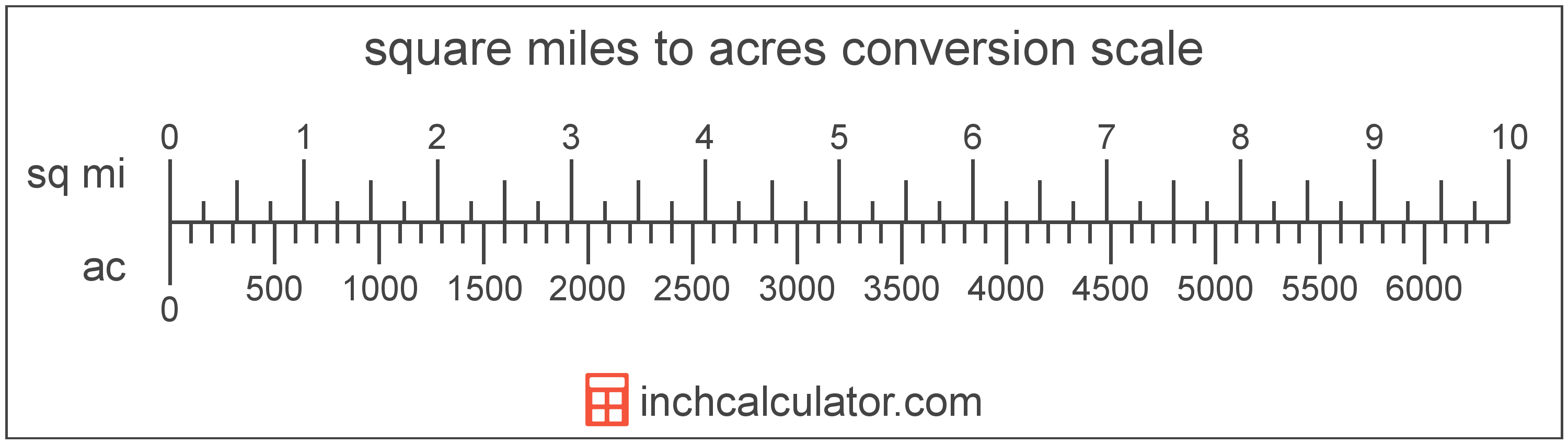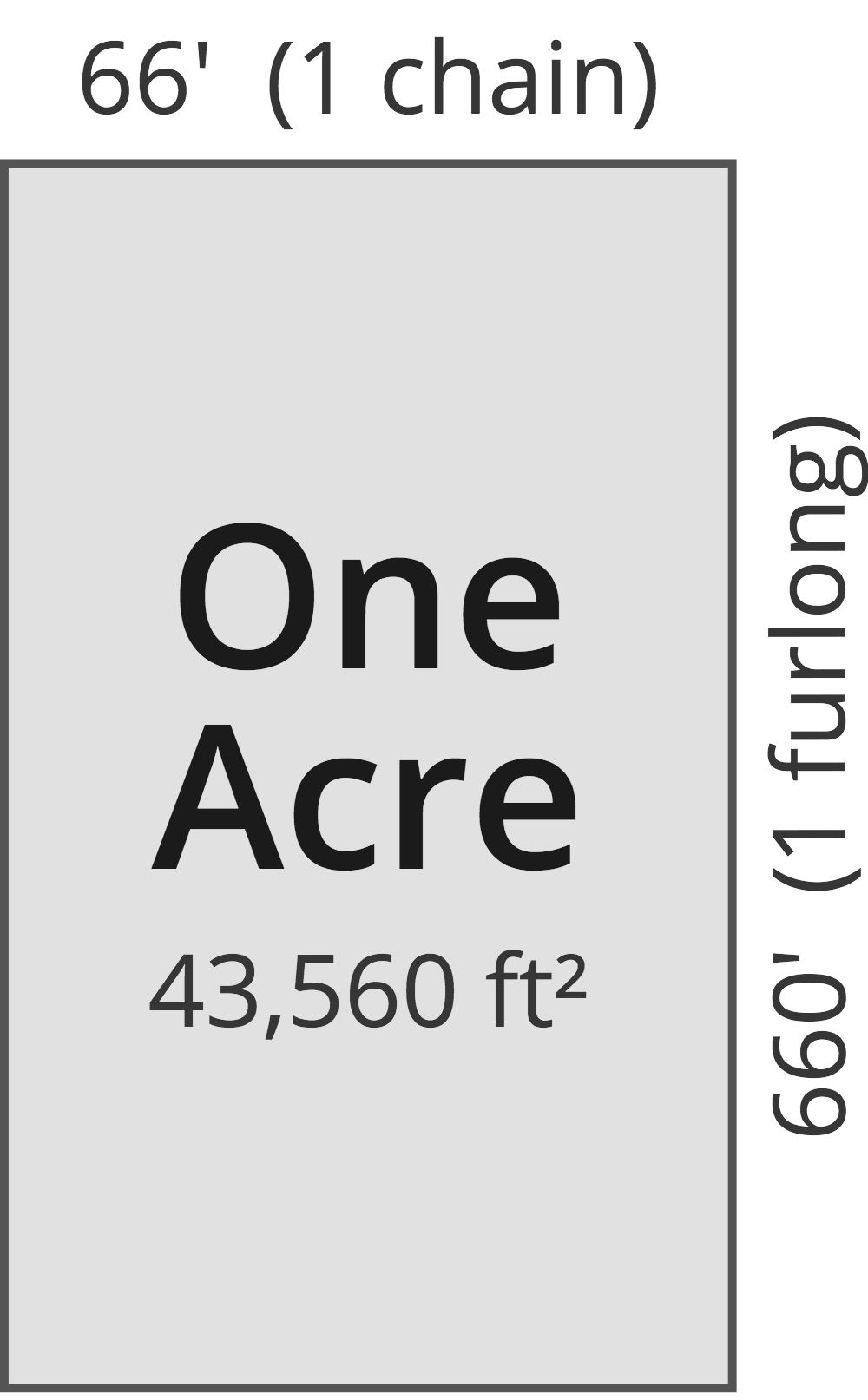# Square Miles to Acres Converter

Enter the area in square miles below to get the value converted to acres.

Results in Acres:1 sq mi = 640 ac

Do you want to convert acres to square miles?

## How to Convert Square Miles to Acres

To convert a measurement in square miles to a measurement in acres, multiply the area by the following conversion ratio: 640 acres/square mile.

Since one square mile is equal to 640 acres, you can use this simple formula to convert:

acres = square miles × 640

The area in acres is equal to the area in square miles multiplied by 640.

For example, here's how to convert 5 square miles to acres using the formula above.
acres = (5 sq mi × 640) = 3,200 ac### How Many Acres Are in a Square Mile?

There are 640 acres in a square mile, which is why we use this value in the formula above.

1 sq mi = 640 ac

## What is a Square Mile?

One square mile is equal to the area of a square with sides that are each 1 mile long. One square mile is roughly equal to 2.59 square kilometers or 640 acres.

The square mile is a US customary and imperial unit of area. Square miles can be abbreviated as sq mi, and are also sometimes abbreviated as mi². For example, 1 square mile can be written as 1 sq mi or 1 mi².

## What is an Acre?

One acre is defined as the area equal to a space that is one chain (66 ft) by one furlong (660 ft), or 10 square chains. That's equal to 43,560 square feet or 1/640 of a square mile for those unfamiliar with those units of measure.The acre is a US customary and imperial unit of area. Acres can be abbreviated as ac; for example, 1 acre can be written as 1 ac.

You can use an acreage calculator to measure the area of a plot of land in acres by locating the boundaries on a map.

## Square Mile to Acre Conversion Table

Table showing various square mile measurements converted to acres.
Square Miles Acres
1 sq mi 640 ac
2 sq mi 1,280 ac
3 sq mi 1,920 ac
4 sq mi 2,560 ac
5 sq mi 3,200 ac
6 sq mi 3,840 ac
7 sq mi 4,480 ac
8 sq mi 5,120 ac
9 sq mi 5,760 ac
10 sq mi 6,400 ac
11 sq mi 7,040 ac
12 sq mi 7,680 ac
13 sq mi 8,320 ac
14 sq mi 8,960 ac
15 sq mi 9,600 ac
16 sq mi 10,240 ac
17 sq mi 10,880 ac
18 sq mi 11,520 ac
19 sq mi 12,160 ac
20 sq mi 12,800 ac
21 sq mi 13,440 ac
22 sq mi 14,080 ac
23 sq mi 14,720 ac
24 sq mi 15,360 ac
25 sq mi 16,000 ac
26 sq mi 16,640 ac
27 sq mi 17,280 ac
28 sq mi 17,920 ac
29 sq mi 18,560 ac
30 sq mi 19,200 ac
31 sq mi 19,840 ac
32 sq mi 20,480 ac
33 sq mi 21,120 ac
34 sq mi 21,760 ac
35 sq mi 22,400 ac
36 sq mi 23,040 ac
37 sq mi 23,680 ac
38 sq mi 24,320 ac
39 sq mi 24,960 ac
40 sq mi 25,600 ac

## References

1. National Institute of Standards and Technology, Specifications, Tolerances, and Other Technical Requirements for Weighing and Measuring Devices, Handbook 44 - 2019 Edition, https://nvlpubs.nist.gov/nistpubs/hb/2019/NIST.HB.44-2019.pdf